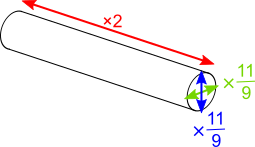#### You may also like### Some(?) of the Parts

A circle touches the lines OA, OB and AB where OA and OB are perpendicular. Show that the diameter of the circle is equal to the perimeter of the triangleA 1 metre cube has one face on the ground and one face against a wall. A 4 metre ladder leans against the wall and just touches the cube. How high is the top of the ladder above the ground?Four rods are hinged at their ends to form a convex quadrilateral. Investigate the different shapes that the quadrilateral can take. Be patient this problem may be slow to load.

# Climbing Ropes

##### Age 14 to 16 ShortChallenge Level

Using three-way scale factors
Compared to the 50 m rope, the 100 m rope is twice as long, and the diameter of the cross-section is 11 rather than 9, which is an increase by a scale factor of $\frac{11}9$.

We can create this by enlarging the 50 m rope in 3 different directions, which are all perpendicular to each other, as shown in this diagram:The weight of the 50 m rope is approximately 2.7 kg, so the weight (in kg) of the 100 m rope is approximately $2.7\times2\times\frac{11}9\times\frac{11}9=2.7\times2\times\frac{121}{81}$

$\frac{121}{81}\approx\frac{120}{80}=\frac32$, so the weight of the 10 m rope is approximately $2.7\times2\times\frac32=2.7\times3=8.1$ kg.

Notice that equivalently, increasing the diameter, a length, by a scale factor of $\frac{11}9$ (without changing the shape of the cross-section) has the effect of increasing the area by a scale factor of $\left(\frac{11}9\right)^2$.

Using formulae
The area of the cross-section of the 50 m rope is $\pi \left(\frac92\right)^2$ square millimetres, and its length is $50000$ mm. So its volume is $50000\times\pi\times\left(\frac92\right)^2$, which means that $50000\times\pi\times\left(\frac92\right)^2\times d=2.7$, where $d$ is the weight of each cubic millimetre of the material the rope is made from (its density).

The 100 m rope is $100 000$ mm long with a cross-sectional area of $\pi \left(\frac{11}2\right)^2$ square millimetres, so its weight will be $100000\times\pi\times\left(\frac92\right)^2\times d$

We can rewrite these to compare them:
50 m rope:          $50 000\times \pi \times 9^2 \div 2^2 = 2.7$
100 m rope: $(2\times50 000)\times\pi \times 11^2\div 2^2 = \hspace{1mm} ?$

So to get from the weight of the 50 m rope to the weight of the 100 m rope, we need to multiply by $2$, divide by $9^2$ and multiply by $11^2$. So the weight of the 100 m rope is approximately: $2.7\times2\times121\div81\approx 2.7\times 2\times (120\div 80)=2.7\times2\times\frac32=2.7\times3=8.1$ kg
You can find more short problems, arranged by curriculum topic, in our short problems collection.